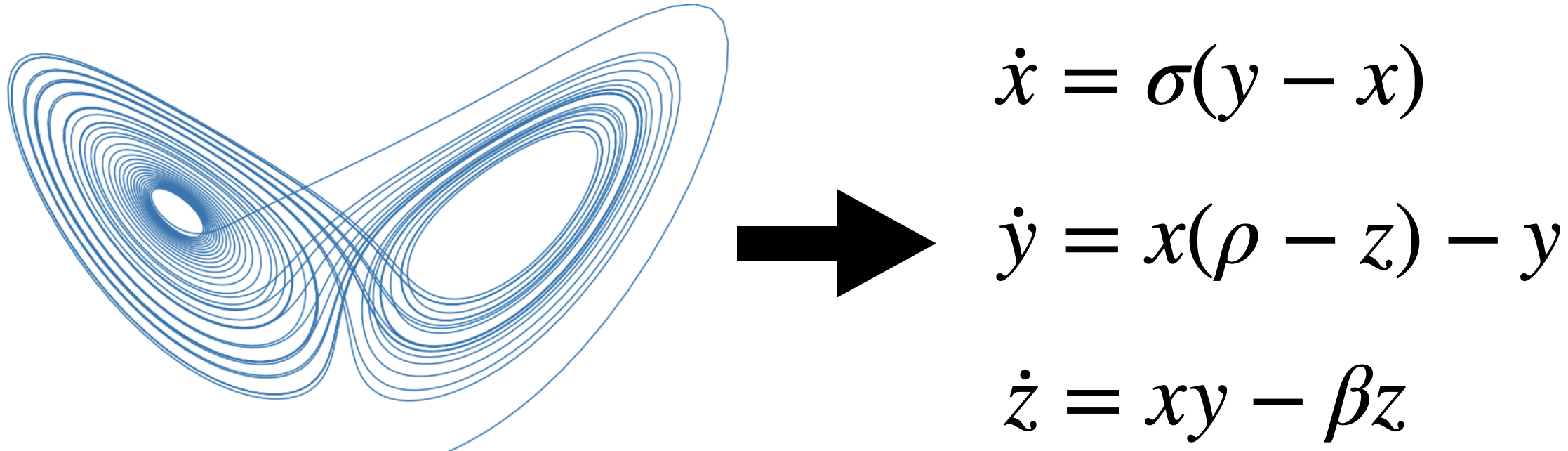# DNN - Tutorial 2 Part I: Physics inspired Machine Learning¶

Sparse Identification of Nonlinear Dynamical Systems (SINDy)

Authors: Leonard Bereska and Ilze Amanda Auzina

This section provides an overview of the Sparse Identification of Nonlinear Dynamical systems (SINDy) algorithm, which was first proposed in:

Brunton, Steven L., Joshua L. Proctor, and J. Nathan Kutz. 2016. “Discovering Governing Equations from Data by Sparse Identification of Nonlinear Dynamical Systems.” Proceedings of the National Academy of Sciences 113 (15): 3932–37. https://doi.org/10.1073/pnas.1517384113.

## What do we mean by identifying nonlinear dynamics from data?¶

Suppose we have time-series data that originate from a (nonlinear) dynamical system.
- To identify a system means to infer the governing equations of that system based on the data. - That is, to find $$f$$ for dynamical systems equations $$\mathbf{\dot x} = f(\mathbf{x})$$ (where $$\mathbf{x}$$ may be vector-valued).For example for the Lorenz system, we want to learn the equations on the right from the time-series data visualized on the left.

## Why do we want sparsity?¶

In this context, by sparse we mean a low number of terms in the governing equations. Sparsity is beneficial, because it is more: 1. Interpretible. Crucial, when understanding the variables and their interactions is needed, e.g. for applications with safety-critical guarantees. 2. Generalizable. If correct, the equations describe the dynamics accurately beyond the region of state space filled by the training data.

In general, one can think of the models identified by SINDy as equations from physics as opposed to big, opaque, deep neural networks.

## SINDy Algorithm¶

SINDy tries to find the dynamical system $$f$$ that suits the data $$\mathrm{\dot X} = f(\mathrm{X})$$. This function approximation problem is formulated as linear regression $$\mathrm{\dot X} = \Theta(\mathrm{X}) \Xi$$, with coefficients $$\Xi$$ and a library of regression terms $$\Theta(X)$$. The algorithm proceeds in three steps:

1. Generate data $$X$$ from a dynamical system and compute derivatives $$\dot X$$.

2. Set up library of candidate terms $$\Theta(X)$$ as functions on $$X$$.

3. Sparsely regress the coefficients $$\Xi$$, that best describe the data.

1. SINDy assumes a time-series of $$n$$-dimensional data points $$\mathbf{x}=(x_1, \ldots x_n)$$ measured at $$m$$ time steps $$t_1, \ldots, t_m$$, we define the data matrix $$X$$ as and derivatives matrix $$\dot X$$:

$\begin{split}X = \begin{bmatrix} x_1(t_1) & x_2(t_1) & \dots & x_n(t_1) \\ x_1(t_2) & x_2(t_2) & \dots & x_n(t_2) \\ \vdots & \vdots & & \vdots \\ x_1(t_m) & x_2(t_m) & \dots & x_n(t_m) \end{bmatrix}, \qquad \dot{X} = \begin{bmatrix} \dot{x_1}(t_1) & \dot{x_2}(t_1) & \dots & \dot{x_n}(t_1) \\ \dot{x_1}(t_2) & \dot{x_2}(t_2) & \dots & \dot{x_n}(t_2) \\ \vdots & \vdots & & \vdots \\ \dot{x_1}(t_m) & \dot{x_2}(t_m) & \dots & \dot{x_n}(t_m) \end{bmatrix}.\end{split}$
1. Next, we define the library matrix $$\Theta(X)$$, the columns of which are a set of basis functions $$\{\theta_l\}_{l=1,\ldots, L}$$ applied to the data:

$\begin{split}\Theta(X) = \begin{bmatrix} \mid & \mid & & \mid \\ \theta_1(X) & \theta_2(X) & \dots & \theta_\ell(X) \\ \mid & \mid & & \mid \end{bmatrix}.\end{split}$

Simple examples are e.g. a basis of polynomials $$x_1, x_2, x_1^2, x_2^2, x_1 x_2, x_1^3 \ldots$$, such as in the Taylor expansion, or trigonometric functions $:nbsphinx-math:sin(x_1), :nbsphinx-math:cos (x_1), :nbsphinx-math:sin(2 x_1), :nbsphinx-math:ldots $, such as in a Fourier expansion. But, depending on the problem more complex basis may be appropriate, e.g. Bessel functions.

1. Lastly, we employ a sparse linear regression algorithm (such as e.g. LASSO) to find the coefficients $$\Xi$$,

$\begin{split}\Xi = \begin{bmatrix} \mid & \mid & & \mid \\ \xi_1 & \xi_2 & \dots & \xi_n \\ \mid & \mid & & \mid \end{bmatrix},\end{split}$

such that

$\mathrm{\dot X} = \Theta(\mathrm{X}) \Xi.$